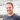Paul Kiddie

# split_str: Split a string based on an array of character delimiters in Matlab

January 14, 2009

I recently needed to do some processing of some data in a text file within matlab where I needed to break up a given string based upon a number of delimiters. I produced the following function which can be used in MATLAB to split a string into a cell array of strings based upon a character array of delimiters:

function parts = split_str(splitstr, str) %split_str Split a string based upon an array of character delimiters % % split_str(splitstr, str) splits the string STR at every occurrence % of an array of characters SPLITSTR and returns the result as a cell % array of strings. % % usage: split_str([_’;’,’],‘hi,there_how,you_doin?‘) % % ans = % % ‘hi’ ‘there’ ‘how’ ‘you’ ‘doin?’ % nargsin = nargin; error(nargchk(2, 2, nargsin));

splitlen = 1; %char’s of length 1 parts = {};

k=[]; %empty array holding indexes of where to split last_split = 1; %index of last split

`for x=1:length(splitstr)      k = \[k strfind(str, splitstr(x))\];     %combines all the found indexes  end.css-1baulvz{display:inline-block;}  k = sort(k);   %sorts out indexes  if isempty(k)     parts{end+1} = str;     return;  end  for x=1:length(k)      parts{end+1} = str(last\_split : k(x)-1);      last\_split = k(x)+1;  end  %now add the final string to the result  parts{end+1} = str(last\_split : length(str));`

end👋 I'm Paul Kiddie, a software engineer working in London. I'm currently working as a Principal Engineer at trainline.